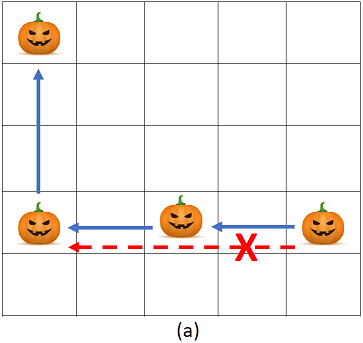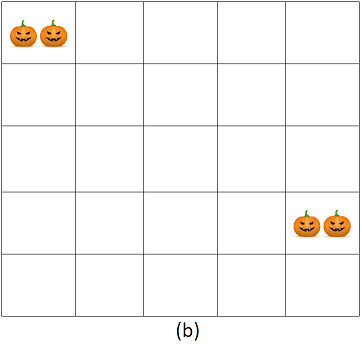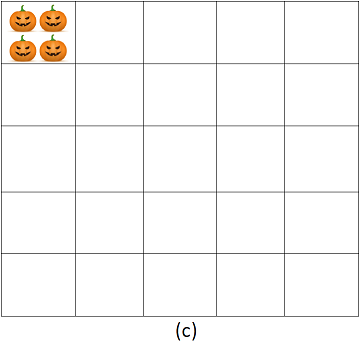### A - A pumpkin game

##### Languages: C, C++, Java, Python, ... (details)

Fito invented a game with pumpkins on a $N \times N$ grid. The aim of the game is to put the pumpkins together. The only possible step in the game is to move one pumpkin to another position that has at least one pumpkin and shares the same column or the same row (moving a pumpkin to a position that doesn't contain any pumpkin is not allowed). Also, all the positions between the pumpkin to be moved and the target positions should be empty. For example, in (a) the movement indicated with the red dashed arrow is not allowed.The game finishes when no pumpkin can be moved and Fito counts then how many positions with pumpkins remain at the end.  For example, for the initial configuration in (a), two possible endings are shown in (b) and (c). Fito wants you to help him compute two values: the (1) minimum and the (2) maximum possible number of positions with pumpkins at the end of the game.#### Input

The input consists of a single test case. The first line contains two integers $N$ and $M$ ($1 \leq N, M \leq 1000$) which are the size of the grid and the number of pumpkins, respectively. Each of the following $M$ lines contains the position of each pumpkin. The $i$-th line contains two integers $x_i$ and $y_i$ that indicate that there is a pumpkin at the intersection of the column $x_i$ and the row $y_i$ ($1 \leq x_i, y_i \leq N$). Initially, there are not two pumpkins in the same position.

#### Output

Print a line with the minimum and the maximum number of positions with pumpkins at the end of the game separated by a single space.

#### Sample test(s)

Input
5 4 1 1 1 4 3 4 5 4
Output
1 2
Input
9 12 2 3 5 8 9 7 9 9 1 2 7 8 9 1 3 3 2 9 4 4 4 6 1 6
Output
3 6
Input
1000 1 1000 1000
Output
1 1